# 跟着官网学习单细胞Monocle3

### 前言

Monocle的官网在：

• 版本2：https://cole-trapnell-lab.github.io/monocle-release/docs/#installing-monocle
• 版本3：https://cole-trapnell-lab.github.io/monocle3/monocle3_docs/

• 处理的细胞数增加很多（millions of cells）
• 针对发育生物学领域，做了一些重大改进：
• 发育轨迹的研究流程优化
• 支持UMAP推断发育轨迹，无缝衔接到`reduceDimension`函数，或者使用独立的函数`UMAP`
• 支持多个祖源（toots）的发育推断
• 利用这个算法approximate graph abstraction 理解各条发育轨迹的分离及平行发育的轨迹
• 3D构建发育轨迹
• 除了发育轨迹以外，官方还介绍它在亚型发现、差异分析方面表现不错
• 另外，版本3在持续不断优化，官方称每几个周就会增加一些新功能
• 其中的算法细节看2019年发表的Cao & Spielmann et al.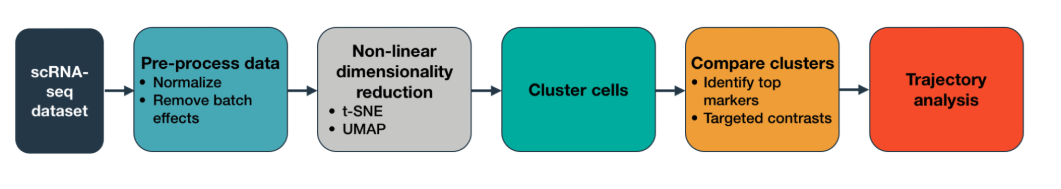Monocle主要分析流程

### 安装版本3

```if (!requireNamespace("BiocManager", quietly = TRUE))
install.packages("BiocManager")
# 一些依赖包
bioc_pkgs <- c('BiocGenerics', 'DelayedArray', 'DelayedMatrixStats',
'limma', 'S4Vectors', 'SingleCellExperiment',
'SummarizedExperiment')
for (bpkg in bioc_pkgs){
if (! require(bpkg,character.only=T) ) {
require(bpkg,character.only=T)
}
}

# (可选)如果要在细胞聚类时设定分辨率参数(resolution)，就要安装一个python包
install.packages("reticulate")
reticulate::py_install("louvain")
# 现在安装3版本，还是要通过github
devtools::install_github('cole-trapnell-lab/monocle3')
# 重启Rstudio，检测是否成功
library(monocle3)
```

### Monocle示例1-细胞聚类及鉴定亚群

#### 创建对象

```cds <- new_cell_data_set(expression_matrix,
```

• 这个对象的灵感来自SingleCellExperiment，因此它的操作也是类似的
• `expression_matrix` 是一个行为基因，列为细胞样本的表达矩阵
• `cell_metadata` 是一个数据框，行为细胞，列是细胞的属性（比如细胞类型、培养环境、培养时间等）
• `gene_metadata`是一个数据框，行为feature信息（比如基因），列是基因属性（比如GC含量）【这个很新鲜，因为其他的基于SingleCellExperiment的包如`Scater` 只需要表达矩阵和样本信息即可】。更重要的是，这个数据框中必须有这么一列：`gene_short_name`，其中保存基因名（简称或Symbol标准名均可，用于作图）
• 并且要满足：`expression_matrix`的行与`gene_metadata`的行对应；`expression_matrix`的列与`cell_metadata`的行对应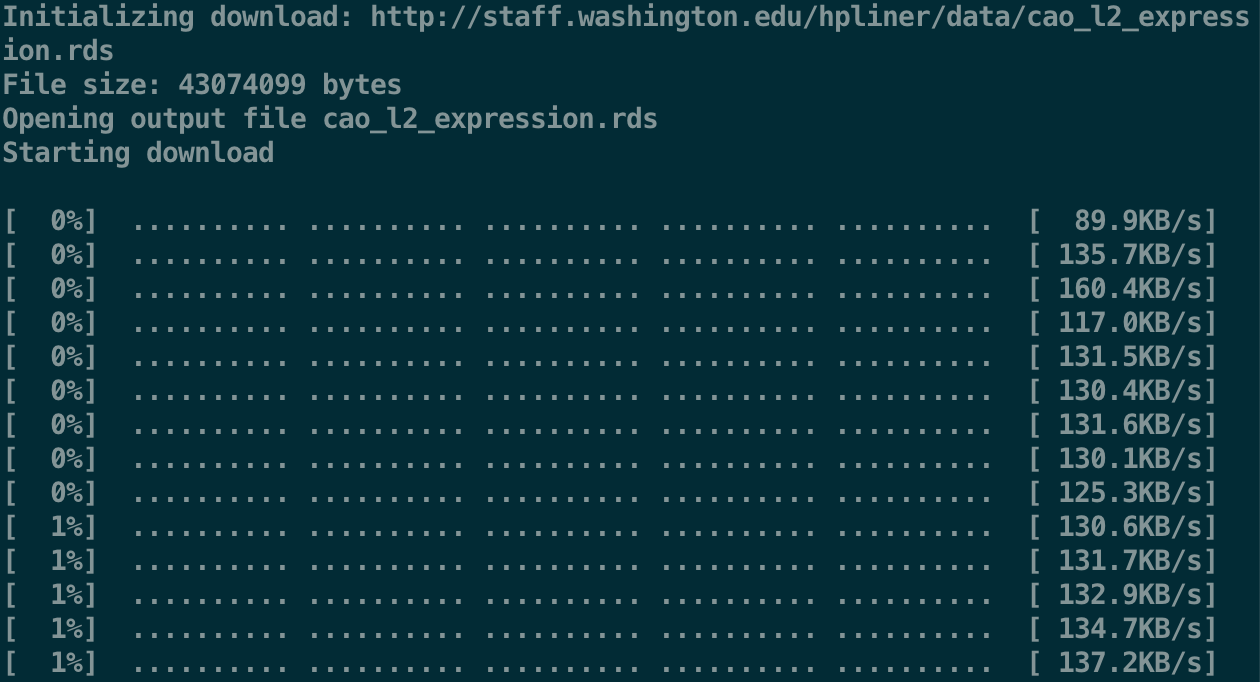```# 下载数据(如果已经有了，可以跳过)

# 加载、探索数据

dim(expression_matrix)
expression_matrix[1:3,1:3]

# 创建CDS(Cell Data Set)对象
cds <- new_cell_data_set(expression_matrix,
cds
```

#### 数据预处理

```# 比较耗时
cds <- preprocess_cds(cds, num_dim = 100) #大约2 mins
# 这里设置这么多主成分，可能是因为细胞数太多(4万多个)，成分太少不足以代表整体
```

• 降维方法`method = c("PCA", "LSI")`，默认是PCA
• 默认维度`num_dim`是50
• 初步降维前归一化`norm_method` 默认是log处理
• 设置降维方法是PCA时，自动进行标准化处理

```plot_pc_variance_explained(cds)
# 很像Seurat的ElbowPlot()
```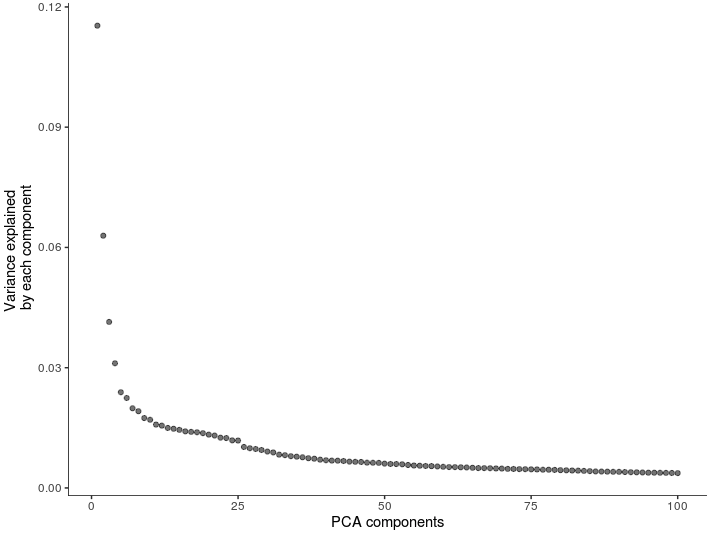#### 继续降维及可视化

（详见`?reduce_dimension`那我就编一个”降维“小故事吧

```cds <- reduce_dimension(cds, preprocess_method = "PCA",reduction_method = c("UMAP"))
# 大约运行1.5 min
```

• 多种继续降维的算法：如`"UMAP", "tSNE", "PCA" and "LSI"`，不过函数默认使用UMAP
• 关于运行加速：
基于`BiocParallel`支持多线程运行，使用`cores`定义；或者使用`umap.fast_sgd=TRUE`参数可以加速降维
但是自己加速的话，它会友情提示一下：

```# Note: reduce_dimension will produce slightly different output each time you run it unless you set 'umap.fast_sgd = FALSE' and 'cores = 1'
```
• 默认得到两个维度
• 需要定义上一步`preprocess_method`使用的算法，默认是`LSI`

```plot_cells(cds)
```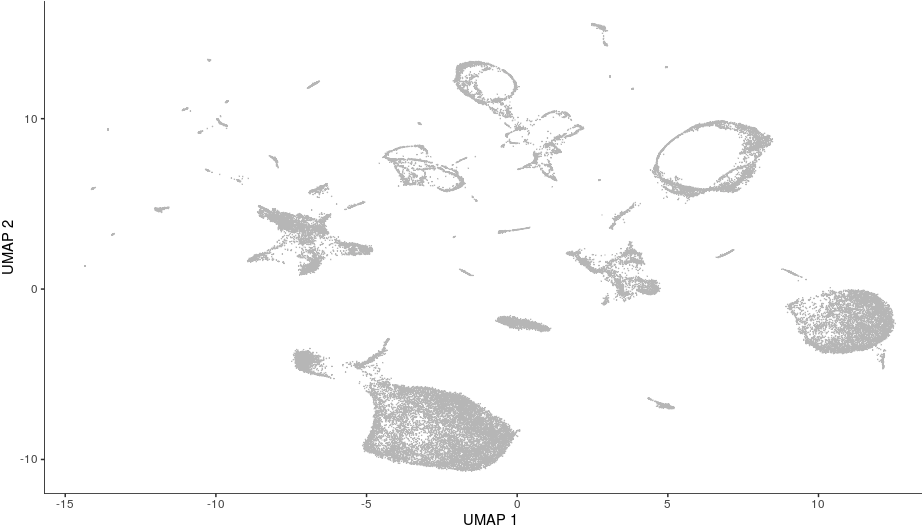```# 数据中一共提供了30类细胞(细胞类型及数量见：)
table(colData(cds)\$"cao_cell_type")
# 根据这个上色
plot_cells(cds, color_cells_by="cao_cell_type")
# (另外除了用cao_cell_type细胞类型，还能用下面的任意一个)
> colnames(colData(cds))
 "plate"         "cao_cluster"   "cao_cell_type" "cao_tissue"    "Size_Factor"
```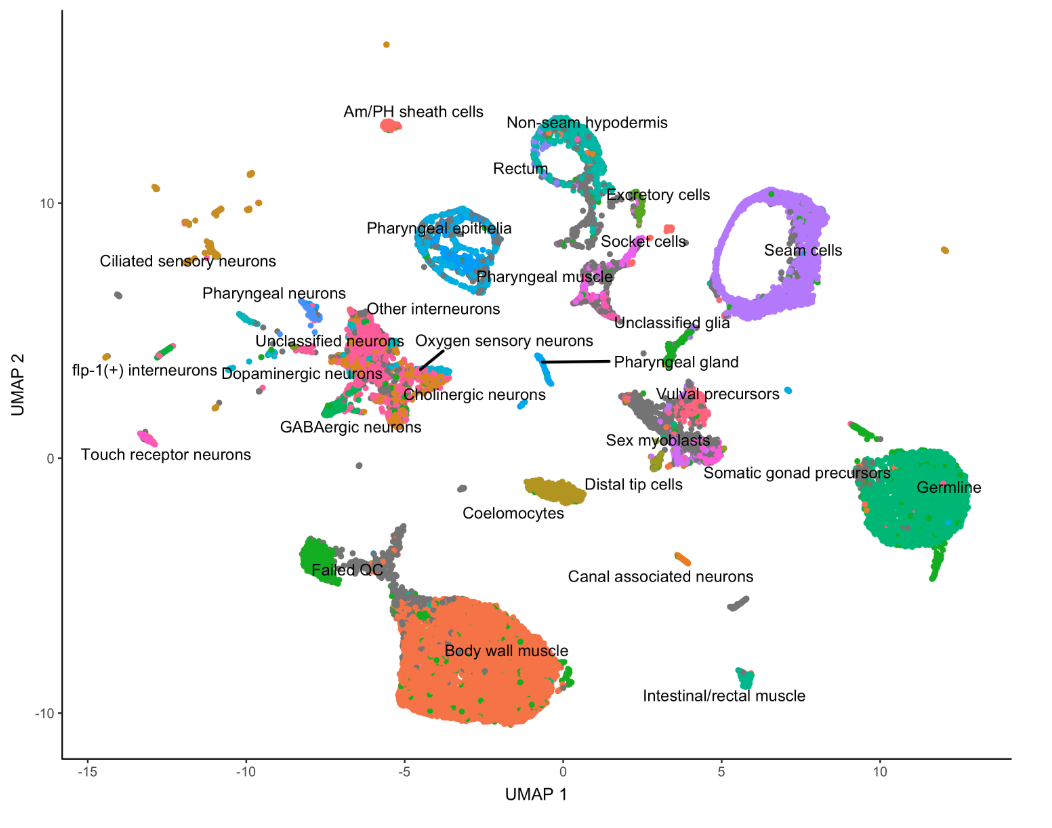```plot_cells(cds, genes=c("cpna-2", "egl-21", "ram-2", "inos-1"))
```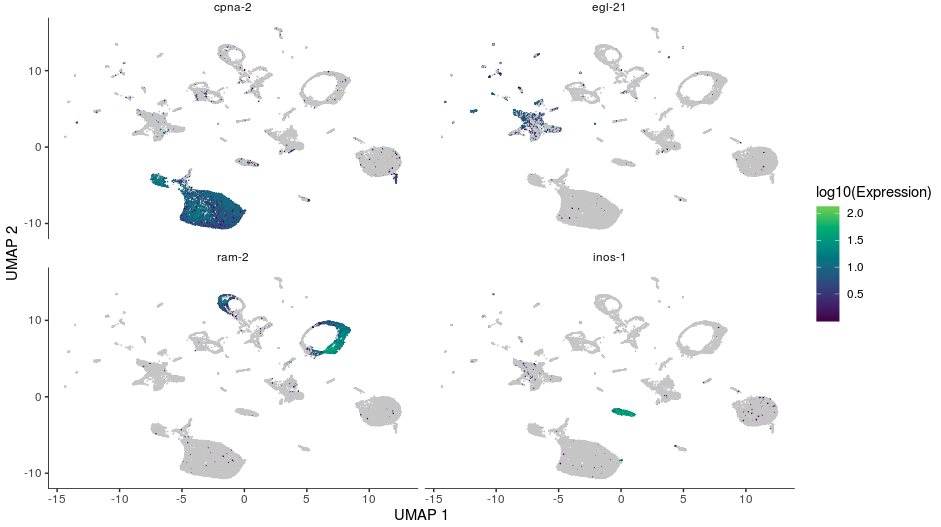```cds <- reduce_dimension(cds, reduction_method="tSNE")
# 然后对tSNE结果可视化
plot_cells(cds, reduction_method="tSNE", color_cells_by="cao_cell_type")
```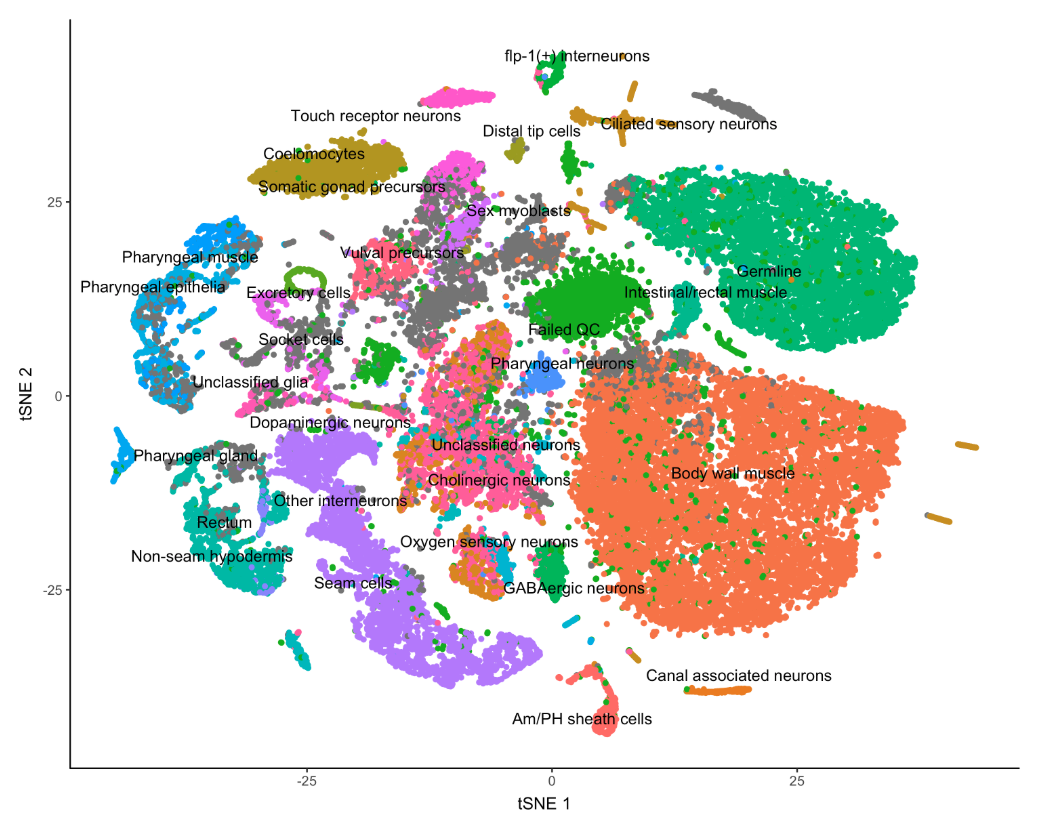image

#### 检查、移除批次效应 => residual_model_formula_str参数

```plot_cells(cds, color_cells_by="plate", label_cell_groups=FALSE)
```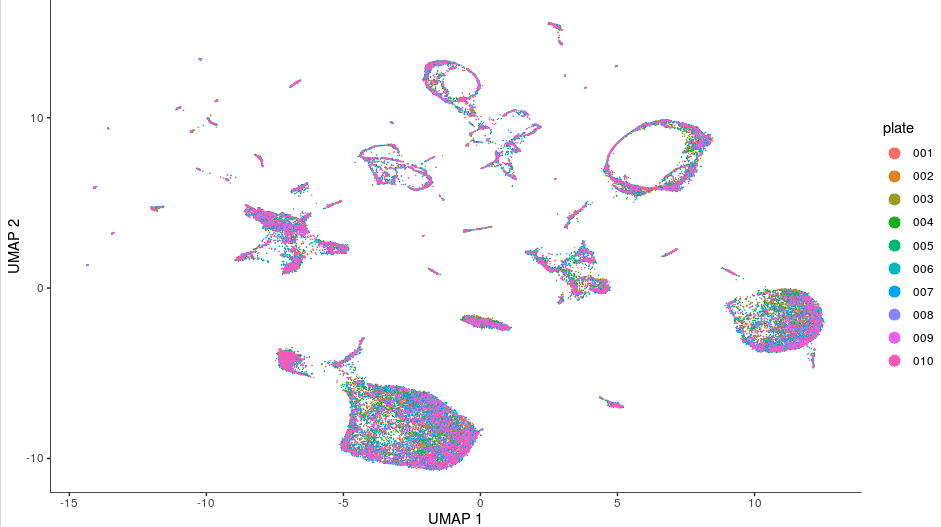```# 假入要对批次进行校正
cds = preprocess_cds(cds, num_dim = 100, residual_model_formula_str = "~ plate")
# 校正后再降维
cds = reduce_dimension(cds)
# 再次检查批次效应
plot_cells(cds, color_cells_by="plate", label_cell_groups=FALSE)
```

#### 细胞聚类 => cluster_cells()

```cds = cluster_cells(cds, resolution=c(10^seq(-6,-1)))
plot_cells(cds)
# 这个可视化函数如果不加任何参数，它默认对不同的cluster上色
# 那么有哪些cluster呢？用下面函数查看：
cds@clusters\$UMAP\$clusters #或者
clusters(cds)
#一共有85个cluster
```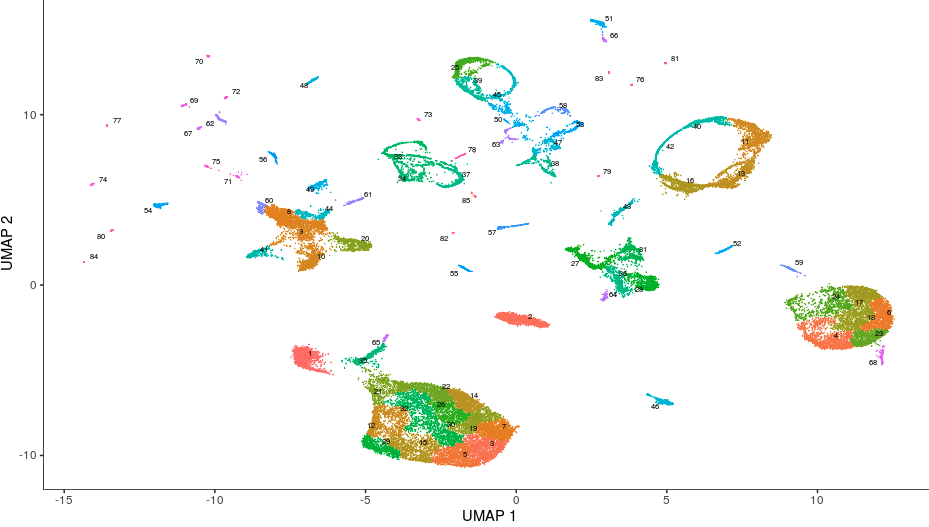```plot_cells(cds, color_cells_by="partition", group_cells_by="partition")
# 检查有多少partition
cds@clusters\$UMAP\$partitions
# 整合成了36个大的partition
```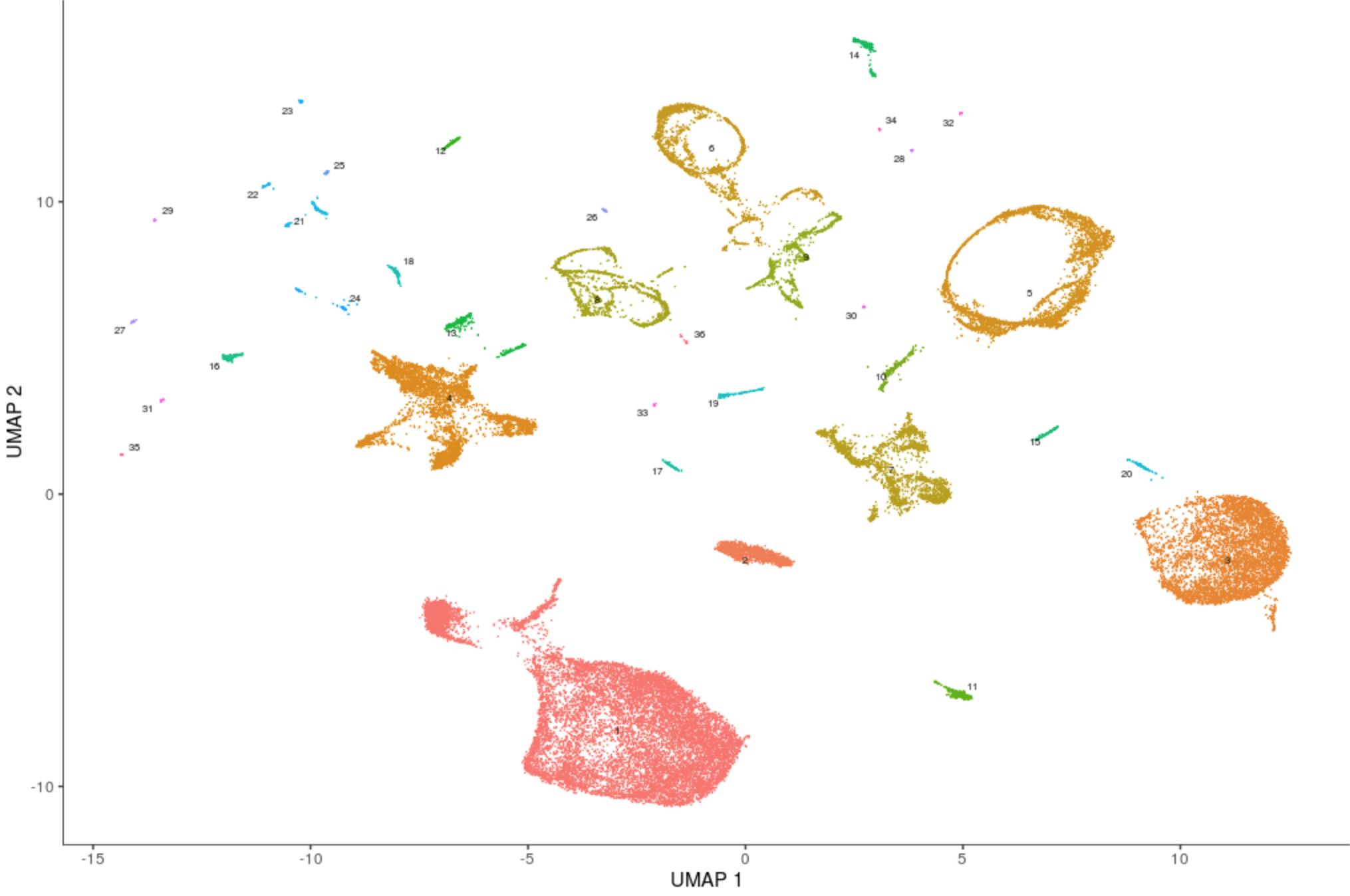```plot_cells(cds, color_cells_by="cao_cell_type")
```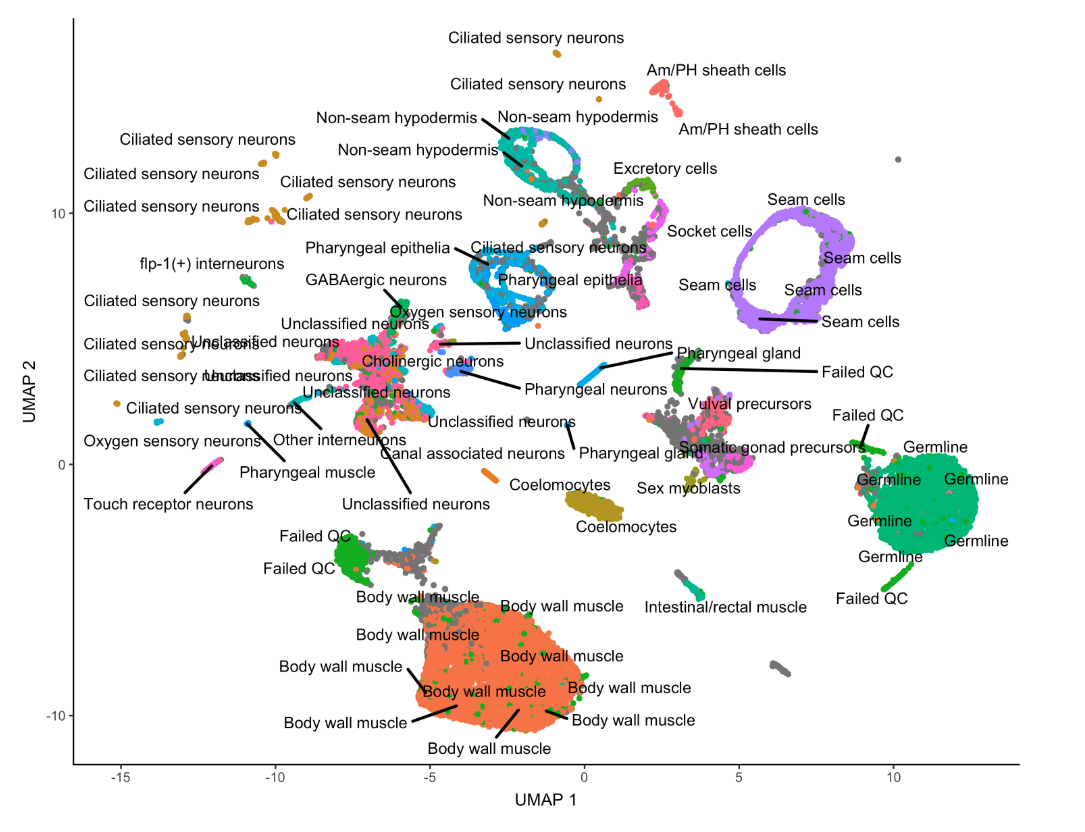```plot_cells(cds, color_cells_by="cao_cell_type", label_groups_by_cluster=FALSE)
```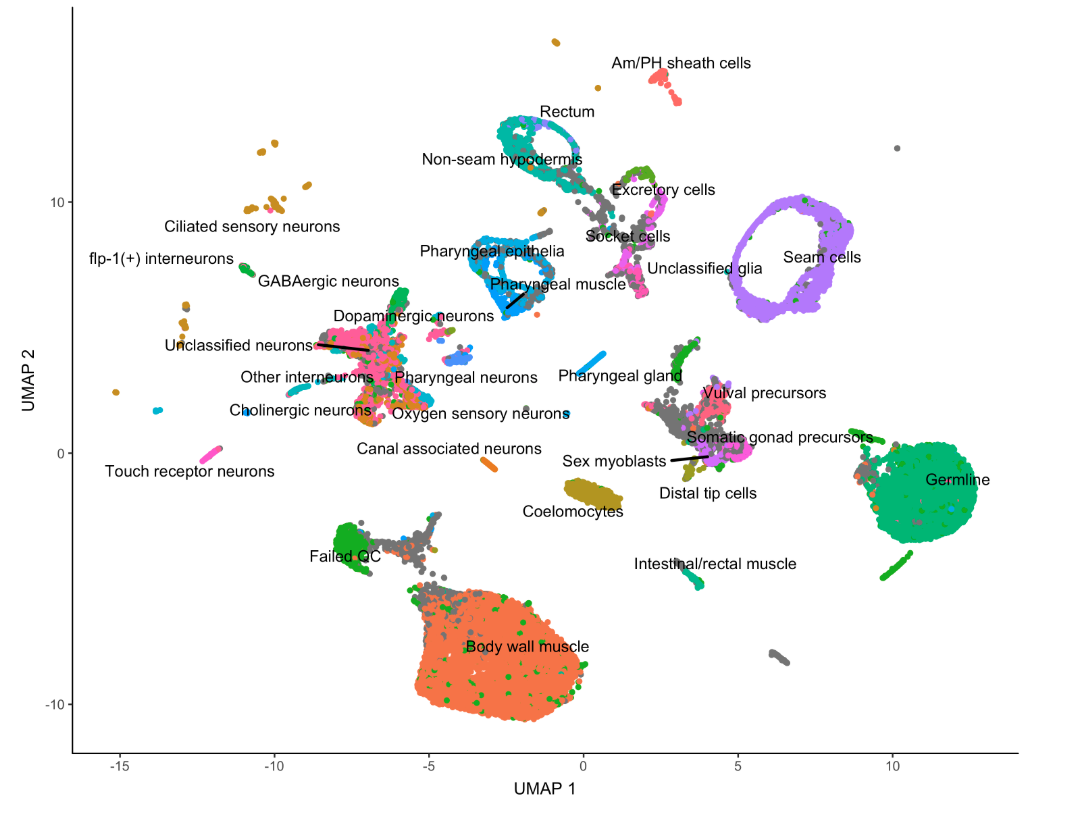#### 找marker基因 => top_markers()

```marker_test_res = top_markers(cds, group_cells_by="partition", reference_cells=1000, cores=8)
# 设置reference_cells是随机挑出来这些数量的细胞作为参照，然后让top_markers和参照集中的基因进行显著性检验；另外reference_cells还可以是来自colnames(cds)的细胞名
marker_test_res[1:4,1:4]
```

```top_specific_markers = marker_test_res %>%
filter(fraction_expressing >= 0.10) %>%
group_by(cell_group) %>%
top_n(1, pseudo_R2)
# 基因id去重
top_specific_marker_ids = unique(top_specific_markers %>% pull(gene_id))
```

```plot_genes_by_group(cds,
top_specific_marker_ids,
group_cells_by="partition",
ordering_type="maximal_on_diag",
max.size=3)
```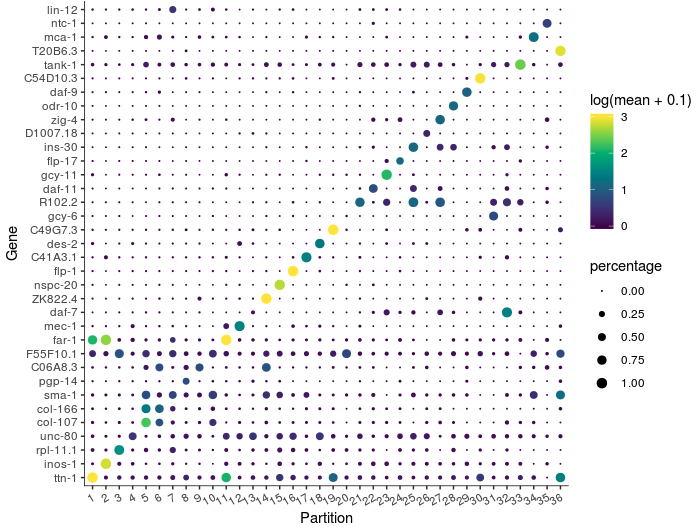```top_specific_markers = marker_test_res %>%
filter(fraction_expressing >= 0.10) %>%
group_by(cell_group) %>%
top_n(3, pseudo_R2)

top_specific_marker_ids = unique(top_specific_markers %>% pull(gene_id))

plot_genes_by_group(cds,
top_specific_marker_ids,
group_cells_by="partition",
ordering_type="cluster_row_col",
max.size=3)
```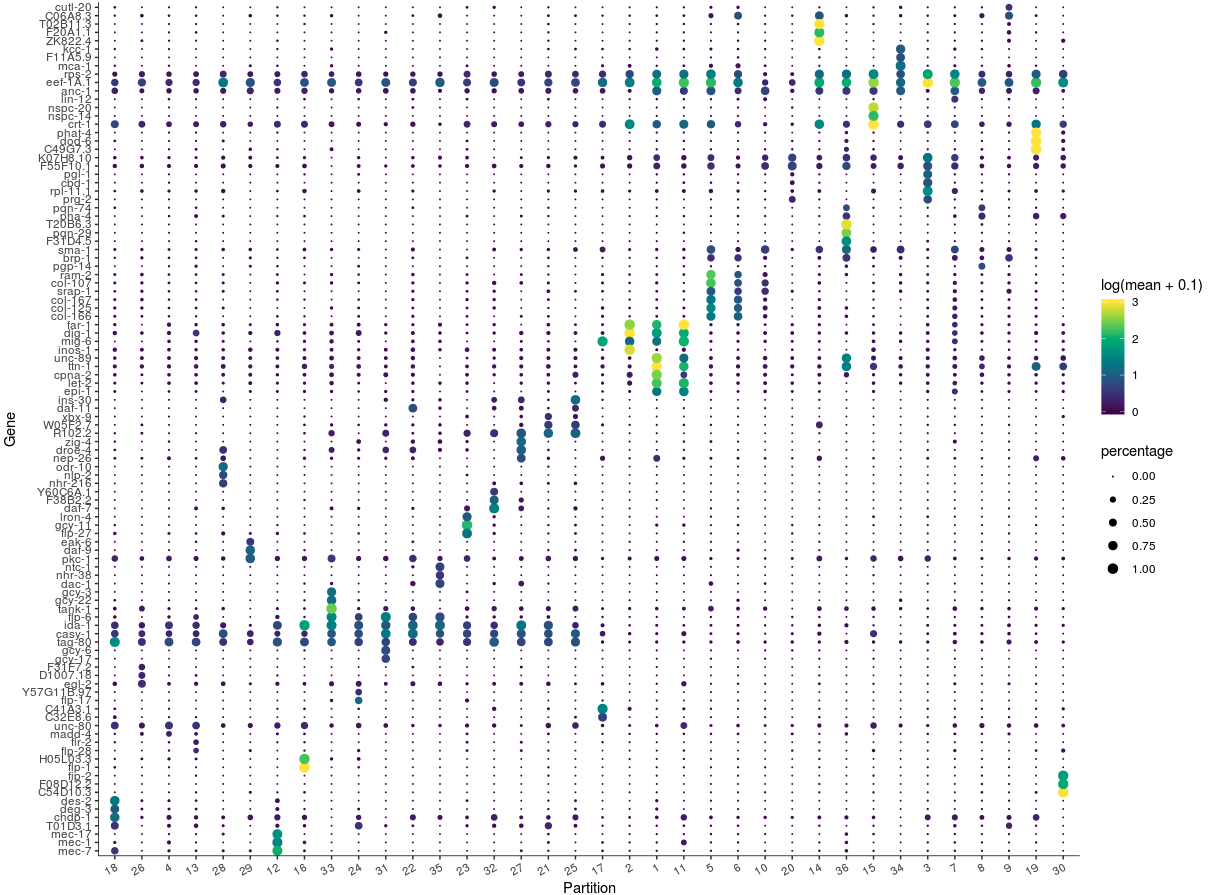#### 对细胞类型进行注释

```# 先将partitions的分组由因子型转为字符型
colData(cds)\$assigned_cell_type = as.character(partitions(cds))
# 再对字符型重新定义
colData(cds)\$assigned_cell_type = dplyr::recode(colData(cds)\$assigned_cell_type,
"1"="Body wall muscle",
"2"="Germline",
"3"="Unclassified neurons",
"4"="Seam cells",
"5"="Coelomocytes",
"6"="Pharyngeal epithelia",
"7"="Vulval precursors",
"8"="Non-seam hypodermis",
"9"="Intestinal/rectal muscle",
"10"="Touch receptor neurons",
"11"="Pharyngeal neurons",
"12"="Am/PH sheath cells",
"13"="NA",
"14"="Unclassified neurons",
"15"="flp-1(+) interneurons",
"16"="Canal associated neurons",
"17"="Pharyngeal gland",
"18"="Other interneurons",
"19"="Ciliated sensory neurons",
"20"="Ciliated sensory neurons",
"21"="Ciliated sensory neurons",
"22"="Ciliated sensory neurons",
"23"="Ciliated sensory neurons",
"24"="Ciliated sensory neurons",
"25"="Oxygen sensory neurons",
"26"="Ciliated sensory neurons",
"27"="Unclassified neurons",
"28"="Pharyngeal gland",
"29"="Ciliated sensory neurons",
"30"="Ciliated sensory neurons",
"31"="Ciliated sensory neurons",
"32"="Ciliated sensory neurons",
"33"="Pharyngeal muscle",
"34"="Failed QC")
plot_cells(cds, group_cells_by="partition", color_cells_by="assigned_cell_type")
# Seurat中用RenameIdents 进行细胞类型重定义

# 另外，想从中取子集、过滤的话
cds[,colData(cds)\$assigned_cell_type != "Failed QC"]
```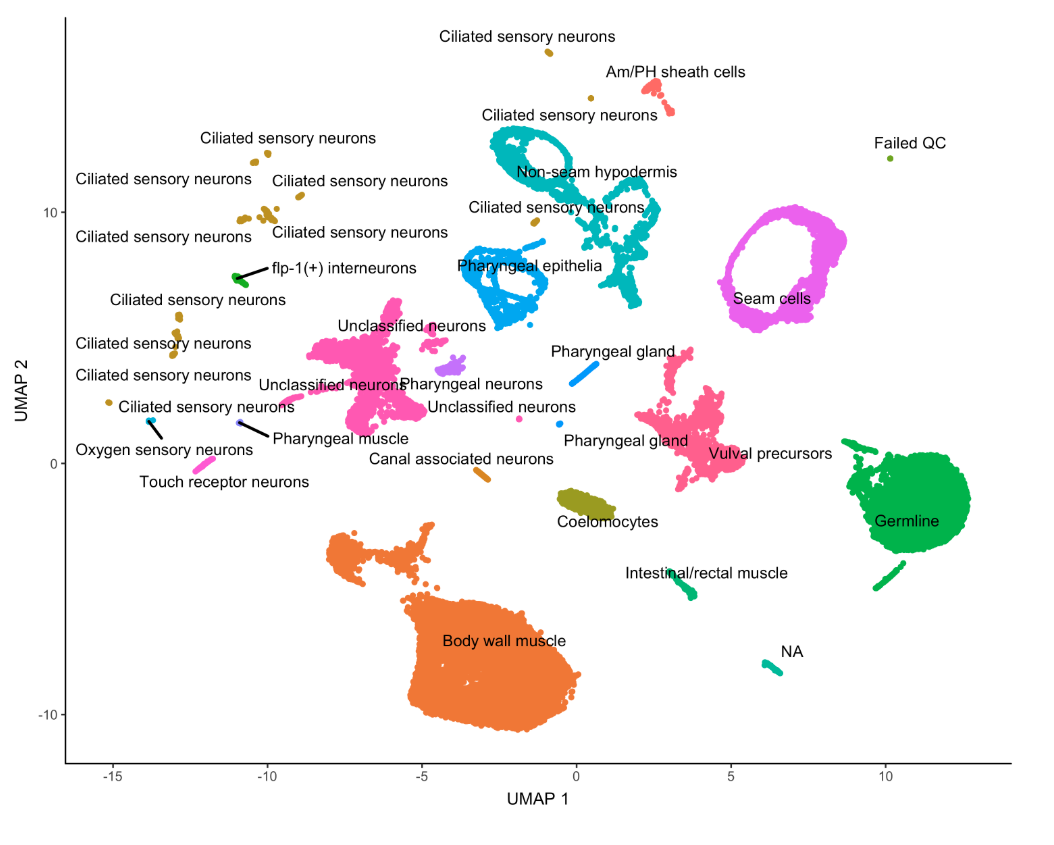#### 基于Garnett的自动化注释

##### 预处理
```# step-1：首先根据上面得到的细胞类型assigned_cell_type，找top_marker
assigned_type_marker_test_res = top_markers(cds,
group_cells_by="assigned_cell_type",
reference_cells=1000,
cores=8)
# step-2：过滤（阈值自定义）
garnett_markers = assigned_type_marker_test_res %>%
filter(marker_test_q_value < 0.01 & specificity >= 0.5) %>%
group_by(cell_group) %>%
top_n(5, marker_score)
# step-3：去重复
garnett_markers = garnett_markers %>% group_by(gene_short_name) %>%
filter(n() == 1)
# step-4：生成marker文件
generate_garnett_marker_file(garnett_markers, file="./marker_file.txt")
```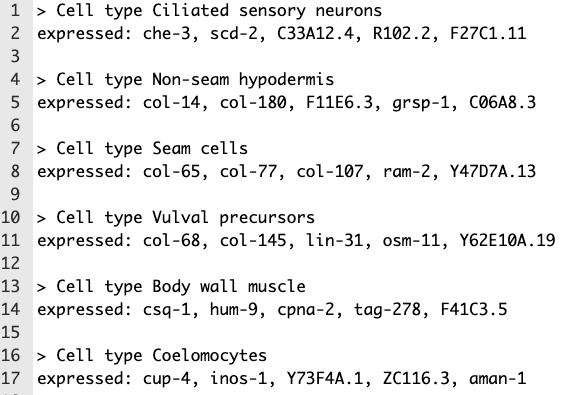generate_garnett_marker_file生成marker文件

##### 方法一：自己使用Garnett
```# 安装Garnett
devtools::install_github("cole-trapnell-lab/garnett", ref="monocle3")
library(garnett)
# 安装物种注释库(这里的物种是C. elegans秀丽隐杆线虫)，目的是为了做基因ID转换
BiocManager::install("org.Ce.eg.db")
colData(cds)\$garnett_cluster = clusters(cds)
worm_classifier <- train_cell_classifier(cds = cds,
marker_file = "./marker_file.txt",
db=org.Ce.eg.db::org.Ce.eg.db,
cds_gene_id_type = "ENSEMBL",
num_unknown = 50,
marker_file_gene_id_type = "SYMBOL",
cores=8)
# 这样自己就做了一个分组数据集，官方也建议将高质量的训练集汇总到：https://github.com/cole-trapnell-lab/garnett/issues
#
cds = classify_cells(cds, worm_classifier,
db = org.Ce.eg.db::org.Ce.eg.db,
cluster_extend = TRUE,
cds_gene_id_type = "ENSEMBL")
plot_cells(cds,
group_cells_by="partition",
color_cells_by="cluster_ext_type")
```
##### 方法二：使用别人提供的分组数据集

```load(ceWhole)
cds = classify_cells(cds, ceWhole,
db = org.Ce.eg.db,
cluster_extend = TRUE,
cds_gene_id_type = "ENSEMBL")
```

### Monocle示例2-构建发育轨迹

#### 载入数据，构建对象 => new_cell_data_set()

```expression_matrix = readRDS(url("http://staff.washington.edu/hpliner/data/packer_embryo_expression.rds"))

# 文件大小(同样可以公众号后台回复"monocle-2"获取)
#|-- [497K]  packer_embryo_colData.rds
#|-- [10.0M]  packer_embryo_expression.rds
#`-- [226K]  packer_embryo_rowData.rds

cds <- new_cell_data_set(expression_matrix,
```

#### 预处理 => preprocess_cds()

```cds <- preprocess_cds(cds, num_dim = 100, residual_model_formula_str = "~ bg.300.loading + bg.400.loading + bg.500.1.loading + bg.500.2.loading + bg.r17.loading + bg.b01.loading + bg.b02.loading")
```

#### 降维可视化 => reduce_dimension()

```cds <- reduce_dimension(cds)
plot_cells(cds, label_groups_by_cluster=FALSE,  color_cells_by = "cell.type")
```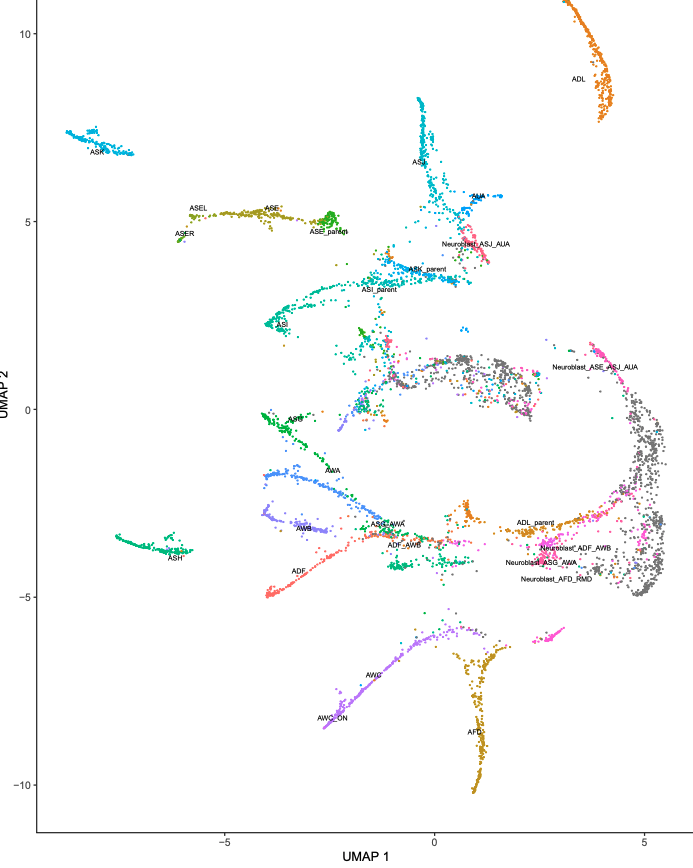```ciliated_genes = c("che-1",
"hlh-17",
"nhr-6",
"dmd-6",
"ceh-36",
"ham-1")

plot_cells(cds,
genes=ciliated_genes,
label_cell_groups=FALSE,
show_trajectory_graph=FALSE)
```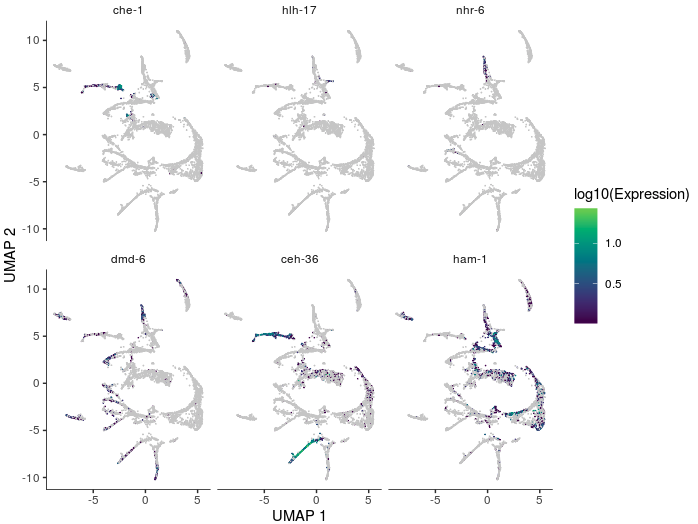image

#### 细胞聚类 => cluster_cells()

Monocle不认为一组数据中的所有细胞都来自同一个”祖先“，很多实验中，它们会有多个发育轨迹。Monocle会通过聚类来判断细胞是否应该归属同一个发育轨迹。之前介绍的`cluster_cells()`中，细胞可以按cluster细分，还可以按partition归为大类。

```# 这里就用partition聚类
cds <- cluster_cells(cds)
plot_cells(cds, color_cells_by = "partition")
```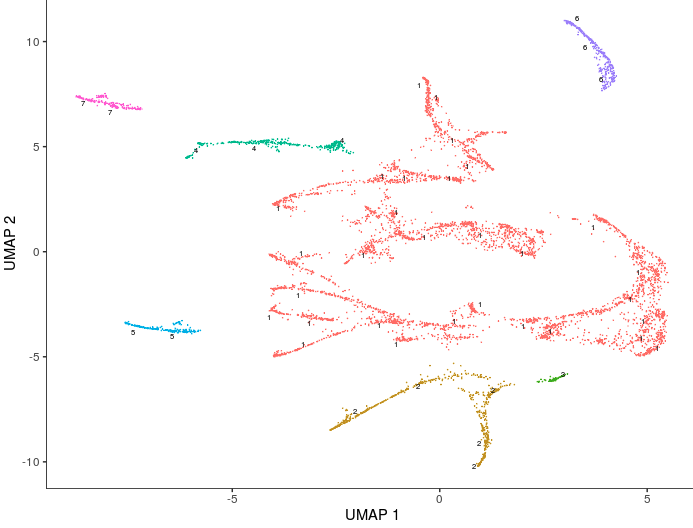#### 利用learn graph在每个partition中寻找主路径 => learn_graph()

```cds <- learn_graph(cds)
plot_cells(cds,
color_cells_by = "cell.type",
label_groups_by_cluster=FALSE,
label_leaves=FALSE,
label_branch_points=FALSE)
```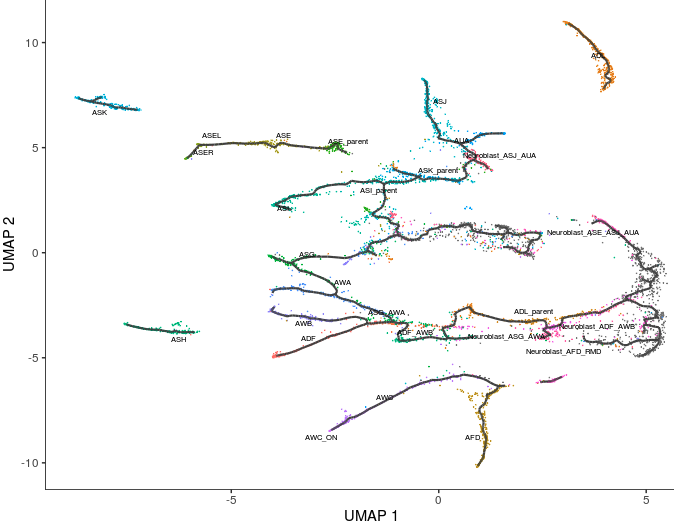#### 有了初步的轨迹线，接下来就是对细胞出现的先后进行排序 => order_cells()

Monocle的这个算法将基因表达量的变化定义为发育轨迹上生命过程的变化（体现为不同的细胞类型），而不是真正的时间变化（因此我们叫它”拟时序“，而不是”真时间分析“）。

```# 先将每个细胞根据”胚胎发育时间区间“进行上色，然后根据胚胎发育过程前后绘制节点
# 在cds@colData中提供了细胞对应的类型以及胚胎发育时间，这个才是真正的关键信息。有了这个信息才能作图。工具不是万能的，它不可能帮助我们去完成生物学中的推断，一定是我们自己先定义好，再交给它进行可视化而已
plot_cells(cds,
color_cells_by = "embryo.time.bin",
label_cell_groups=FALSE,
label_leaves=TRUE,
label_branch_points=TRUE,
graph_label_size=1.5)
```• 图中的黑线就是整个架构（注意到整个图并非完全连接的，毕竟是按照partition聚类，每个partition中的细胞差异有点大）；
• 浅灰色的圆圈表示”叶片“表示发育轨迹中的不同结局（可以认为拟时序分析的图是一个”根-茎-叶“结构），用参数`label_leaves`控制；
• 黑色的圆圈表示分支节点，预示着其中的细胞会有不同发展方向，用参数`label_branch_points`控制；
• 圈中数字大小表示出现时间的先后

```# 官方给出了一个函数，这里定义了一个time_bin，选择了最早的时间点区间。
get_earliest_principal_node <- function(cds, time_bin="130-170"){
# 首先找到出现在最早时间区间的细胞ID
cell_ids <- which(colData(cds)[, "embryo.time.bin"] == time_bin)

closest_vertex <-
cds@principal_graph_aux[["UMAP"]]\$pr_graph_cell_proj_closest_vertex
closest_vertex <- as.matrix(closest_vertex[colnames(cds), ])
root_pr_nodes <-
igraph::V(principal_graph(cds)[["UMAP"]])\$name[as.numeric(names
(which.max(table(closest_vertex[cell_ids,]))))]

root_pr_nodes
}
cds = order_cells(cds, root_pr_nodes=get_earliest_principal_node(cds))
```

```plot_cells(cds,
color_cells_by = "pseudotime",
label_cell_groups=FALSE,
label_leaves=FALSE,
label_branch_points=FALSE,
graph_label_size=1.5)
```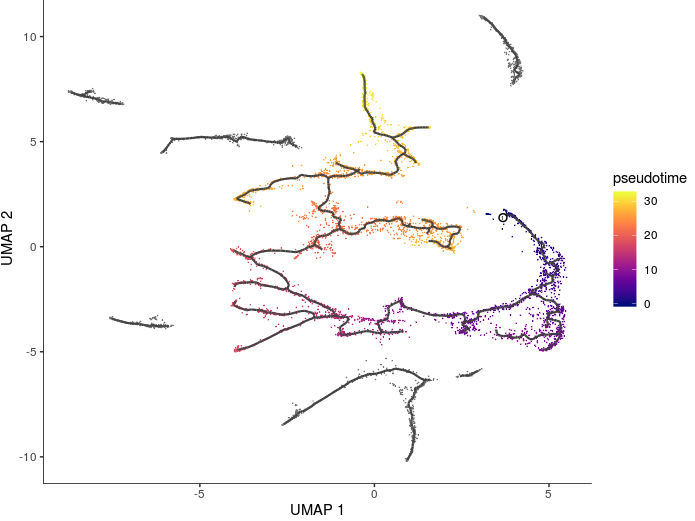#### 利用3D的发育轨迹对上述内容做个概述

3D轨迹实际上就是降维时选前3个主成分 => `max_components = 3`，后续都和2D保持类似

```# 3D trajectories（4步走）
cds_3d = reduce_dimension(cds, max_components = 3)
cds_3d = cluster_cells(cds_3d)
cds_3d = learn_graph(cds_3d)
cds_3d = order_cells(cds_3d, root_pr_nodes=get_earliest_principal_node(cds))

cds_3d_plot_obj = plot_cells_3d(cds_3d, color_cells_by="partition")
cds_3d_plot_obj
```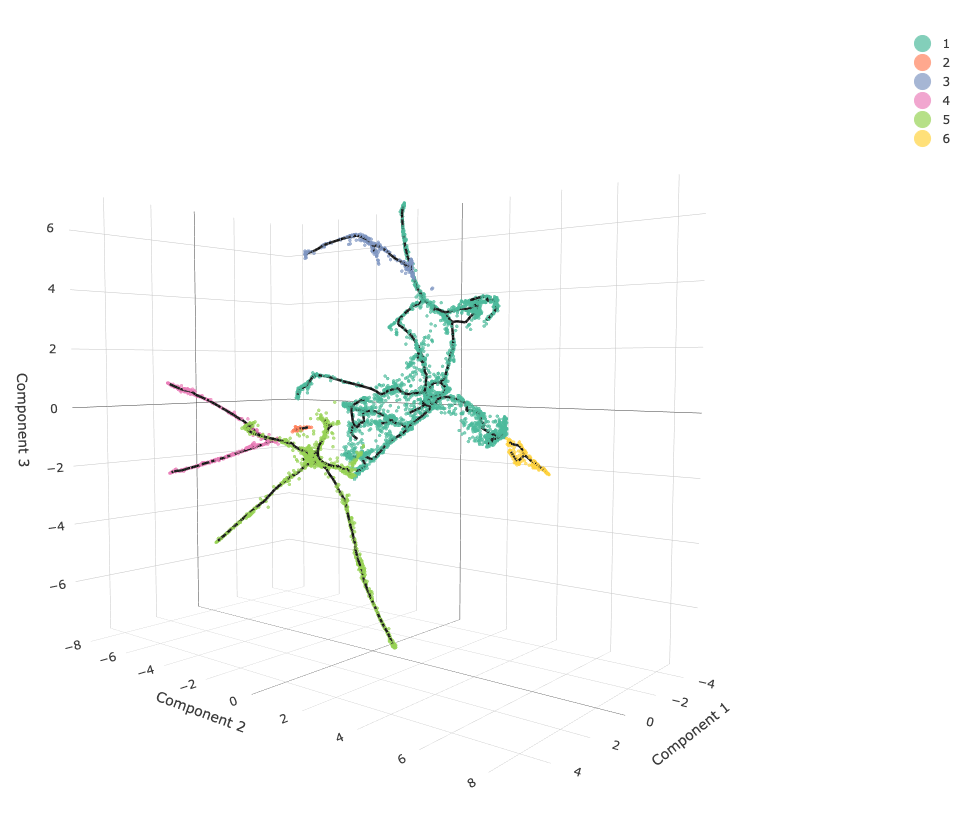3D发育轨迹

### Monocle差异分析

Monocle3提供了不同细胞类型之间寻找差异基因的方法，主要有两种：

• Regression analysis：利用`fit_models()`，用来评价基因表达是否会受到诸如时间、处理等的影响
• Graph-autocorrelation analysis：利用`graph_test()`，用来寻找一条轨迹或不同cluster中基因的差异

#### 方法一：Regression analysis => fit_models()

```ciliated_genes = c("che-1",
"hlh-17",
"nhr-6",
"dmd-6",
"ceh-36",
"ham-1")
cds_subset = cds[rowData(cds)\$gene_short_name %in% ciliated_genes,]
```

```gene_fits = fit_models(cds_subset, model_formula_str = "~embryo.time")
# 其中model_formula_str就是要比较的分组对象，如果相获得不同的cluster或者partition的差异基因，就用model_formula_str = "~cluster"或者model_formula_str = "~partition"；另外还支持添加多个变量，比如考虑到批次效应 model_formula_str = "~embryo.time + batch"
```

```fit_coefs = coefficient_table(gene_fits)
# 挑出时间相关的组分
emb_time_terms = fit_coefs %>% filter(term == "embryo.time")
# coefficient_table()默认使用 Benjamini and Hochberg（BH）方法进行了p值的校正，得到了q值
emb_time_terms %>% filter (q_value < 0.05) %>%
select(gene_short_name, term, q_value, estimate)

# 结果数据集中的6个基因有5个是时间相关的差异基因
```

```plot_genes_violin(cds_subset, group_cells_by="embryo.time.bin", ncol=2) +
theme(axis.text.x=element_text(angle=45, hjust=1))
```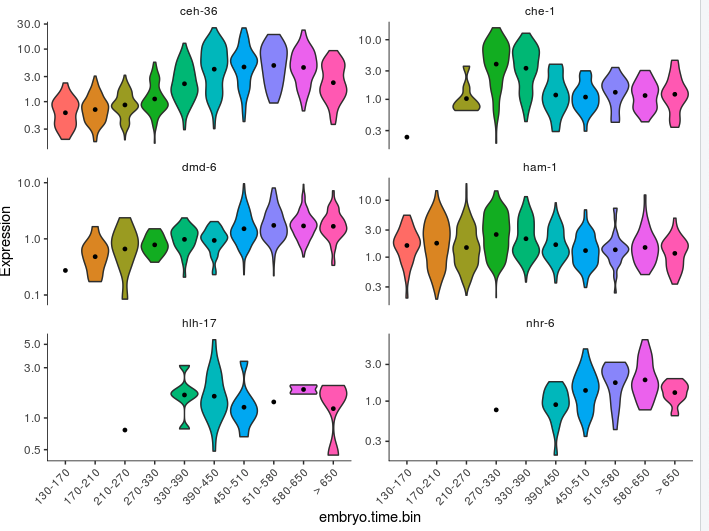##### 如果要考虑批次效应
```gene_fits = fit_models(cds_subset, model_formula_str = "~embryo.time + batch")
fit_coefs = coefficient_table(gene_fits)
fit_coefs %>% filter(term != "(Intercept)") %>%
select(gene_short_name, term, q_value, estimate)
```
##### 模型做出来了，效果到底如何？ => evaluate_fits()

```gene_fits = fit_models(cds_subset, model_formula_str = "~embryo.time")
evaluate_fits(gene_fits)
```

```time_batch_models = fit_models(cds_subset,
model_formula_str = "~embryo.time + batch",
expression_family="negbinomial")
time_models = fit_models(cds_subset,
model_formula_str = "~embryo.time",
expression_family="negbinomial")
compare_models(time_batch_models, time_models) %>% select(gene_short_name, q_value)
```

```gene_short_name  q_value
<chr>              <dbl>
1 ham-1           3.74e-20
2 dmd-6           7.11e-37
3 hlh-17          1.04e- 5
4 nhr-6           5.95e- 3
5 ceh-36          6.23e-10
6 che-1           5.06e-6
```

`fit_models()`函数支持多种分布，默认是：`quasipoisson` ，它和负二项分布相似，只不过比负二项分布准确性低一点、速度会更快，对于细胞数量多更适用。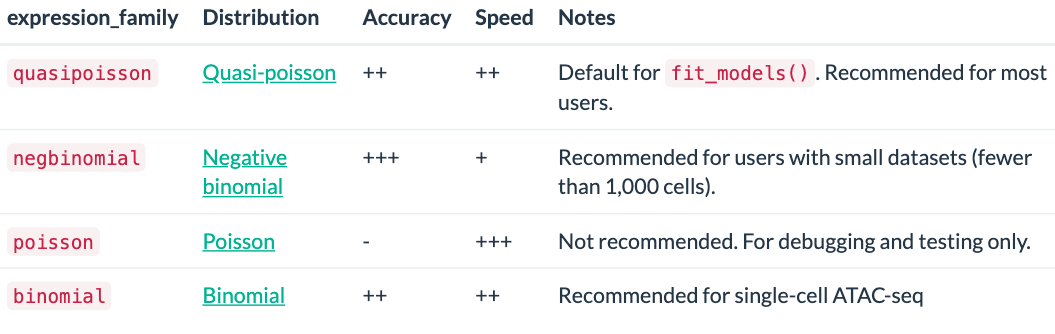#### 方法二：Graph-autocorrelation analysis => graph_test()

```# 选一部分神经元细胞
neurons_cds = cds[,colData(cds)\$assigned_cell_type == "Neurons"]
plot_cells(neurons_cds, color_cells_by="partition")
```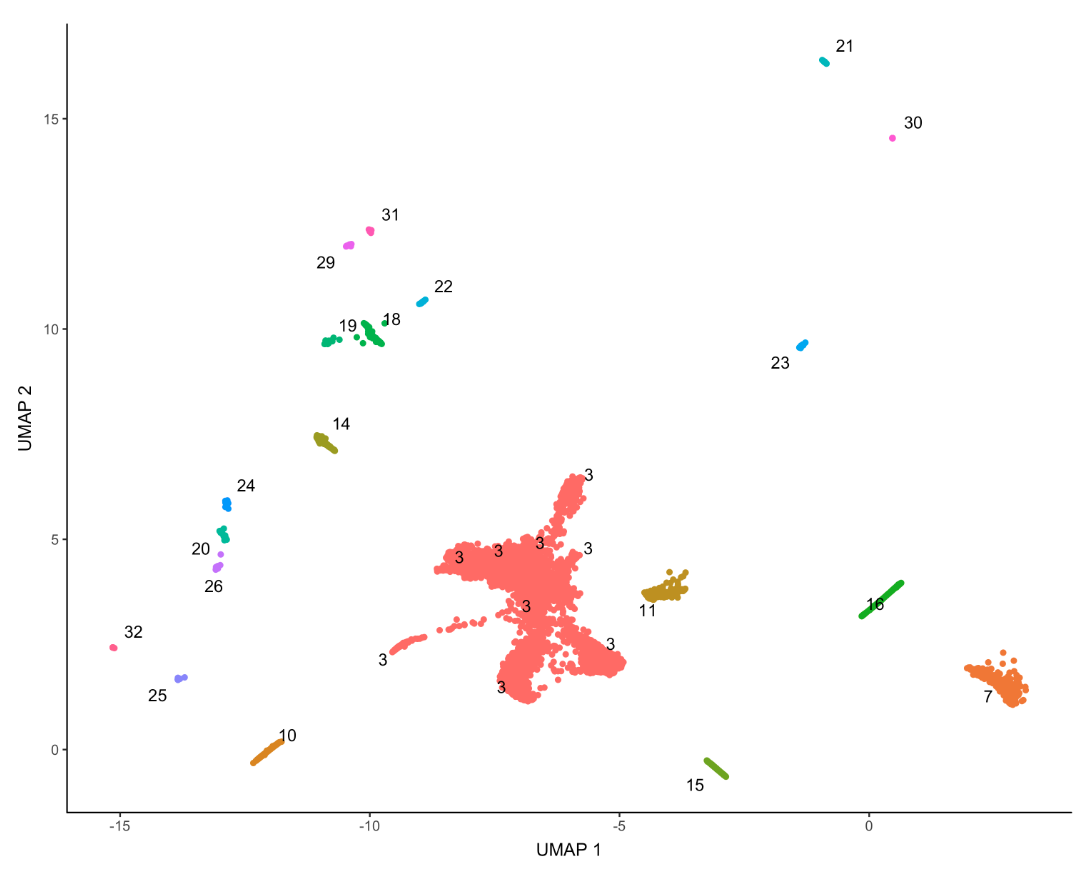`Moran's I`这个指标叫：莫兰指数，澳大利亚统计学家莫兰于1950年提出。它是用来度量空间相关性的一个指标，数据经过方差归一化之后，会落在-1.0和1.0之间。这个值大于0表示空间正相关，值越大空间相关性越强；等于0时空间是随机性分布；小于0时表示空间负相关，值越小空间差异越大

```pr_graph_test_res = graph_test(neurons_cds, neighbor_graph="knn", cores=8)
pr_deg_ids = row.names(subset(pr_graph_test_res, q_value < 0.05))
```

`pr_graph_test_res`结果是一个数据框，存储了cds对象中每个基因的`Moran's I`检验结果。正值表示基因分布在UMAP空间的一小块”焦点“区域（例如一个或几个cluster）。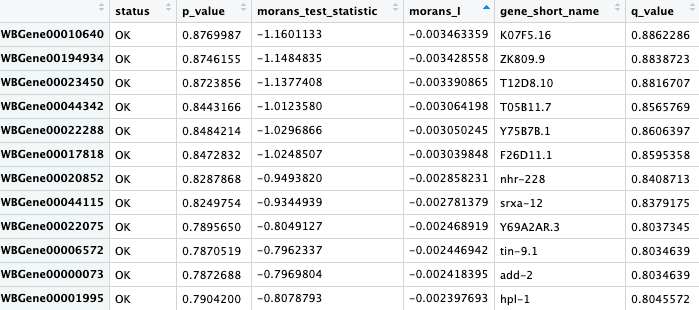pr_graph_test_res结果

#### 将共同作用的基因合并成模块 => find_gene_modules()

```gene_module_df = find_gene_modules(neurons_cds[pr_deg_ids,], resolution=1e-2)
```

• 第一种：
```cell_group_df = tibble::tibble(cell=row.names(colData(neurons_cds)), cell_group=partitions(cds)[colnames(neurons_cds)])
agg_mat = aggregate_gene_expression(neurons_cds, gene_module_df, cell_group_df)
row.names(agg_mat) = stringr::str_c("Module ", row.names(agg_mat))
colnames(agg_mat) = stringr::str_c("Partition ", colnames(agg_mat))

pheatmap::pheatmap(agg_mat, cluster_rows=TRUE, cluster_cols=TRUE,
scale="column", clustering_method="ward.D2",
fontsize=6)
```

结果大体是这样：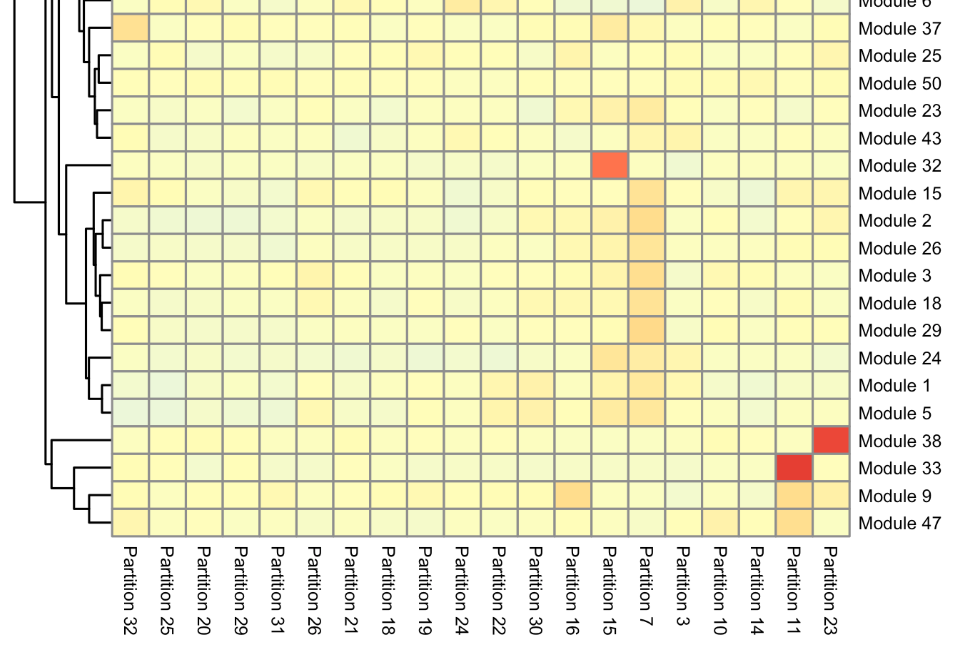• 第二种：针对少数量的module可以看得比较清楚，这里选了4个
```plot_cells(neurons_cds,
genes=gene_module_df %>% filter(module %in% c(16,38,33,42)),
group_cells_by="partition",
color_cells_by="partition",
show_trajectory_graph=FALSE)
```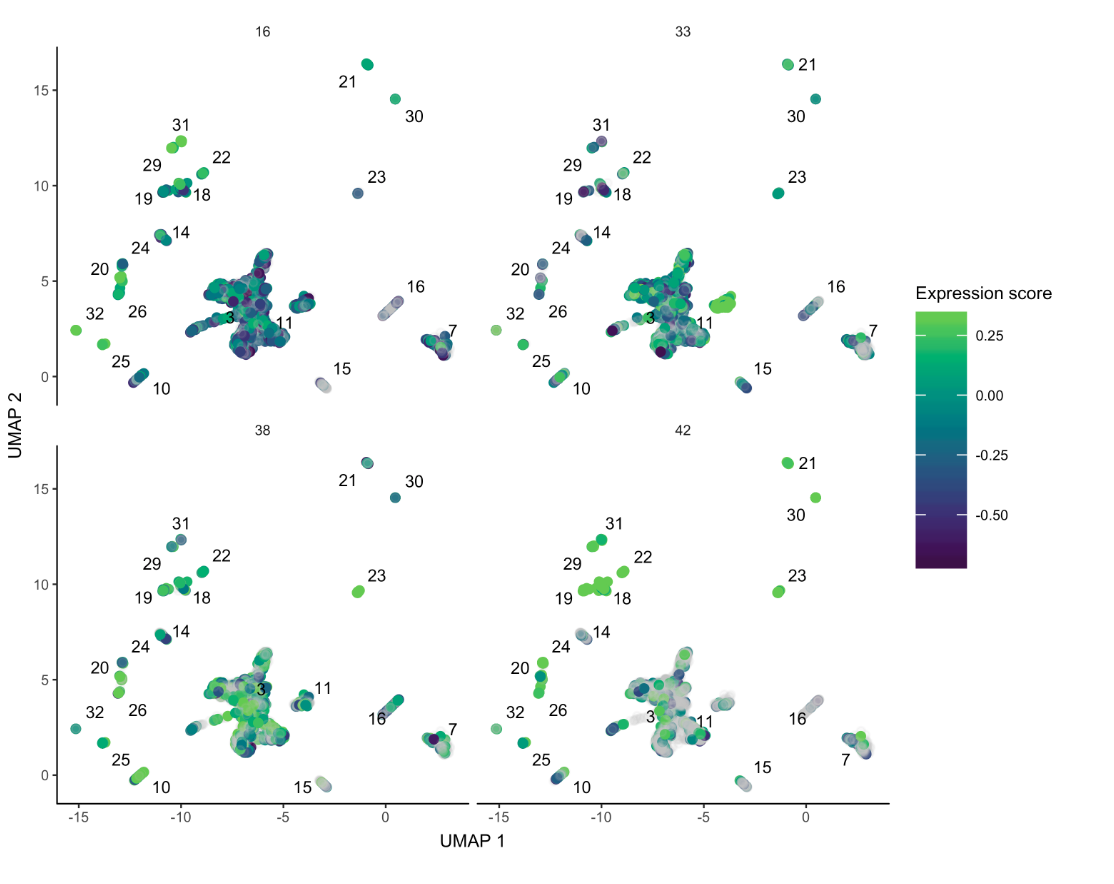#### 找到影响发育轨迹的基因

```ciliated_cds_pr_test_res = graph_test(cds, neighbor_graph="principal_graph", cores=4)
# 使用neighbor_graph="principal_graph"来检验轨迹相邻的细胞的表达是否相关
pr_deg_ids = row.names(subset(ciliated_cds_pr_test_res, q_value < 0.05))
# 从pr_deg_ids中挑选几个基因，然后可视化
plot_cells(cds, genes=c("hlh-4", "gcy-8", "dac-1", "oig-8"),
show_trajectory_graph=FALSE,
label_cell_groups=FALSE,
label_leaves=FALSE)

# 可以将这些在轨迹上变化的pr_deg_ids继续分为小模块
gene_module_df = monocle3:::find_gene_modules(cds[pr_deg_ids,], resolution=c(0,10^seq(-6,-1)))

cell_group_df = tibble::tibble(cell=row.names(colData(cds)), cell_group=colData(cds)\$cell.type)
agg_mat = aggregate_gene_expression(cds, gene_module_df, cell_group_df)
row.names(agg_mat) = stringr::str_c("Module ", row.names(agg_mat))
pheatmap::pheatmap(agg_mat,
scale="column", clustering_method="ward.D2")

plot_cells(cds,
genes=gene_module_df %>% filter(module %in% c(29,20, 11,22)),
label_cell_groups=FALSE,
show_trajectory_graph=FALSE)
```
##### 另外还有一种方法：

```plot_cells(cds, show_trajectory_graph=FALSE)
```

```# 假设这里AFD细胞对应一段22、28、35组成的轨迹线
AFD_genes = c("gcy-8", "dac-1", "oig-8")
AFD_lineage_cds = cds[rowData(cds)\$gene_short_name %in% AFD_genes,
clusters(cds) %in% c(22, 28, 35)]

plot_genes_in_pseudotime(AFD_lineage_cds,
color_cells_by="embryo.time.bin",
min_expr=0.5)
```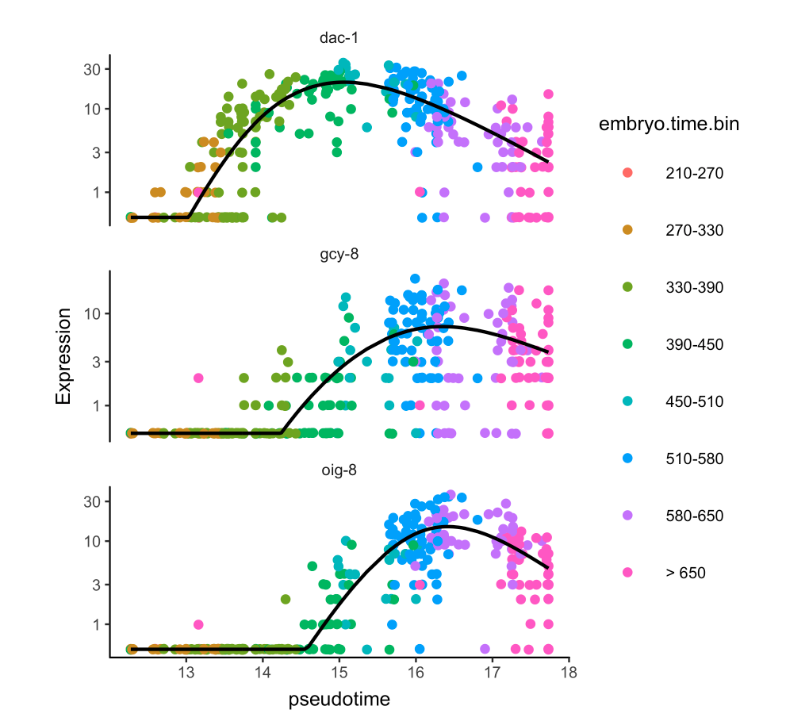### 如果使用版本2...

• 需要指定`exprs``phenoData``featureData`，后两个都要是`AnnotatedDataFrame`对象（目的是不让用户随便修改，看来还是版本3比较人性化），然后依然是要与表达矩阵行、列对应
```#do not run

pd <- new("AnnotatedDataFrame", data = HSMM_sample_sheet)
fd <- new("AnnotatedDataFrame", data = HSMM_gene_annotation)
HSMM <- newCellDataSet(as.matrix(HSMM_expr_matrix),
phenoData = pd, featureData = fd)
```

### 体验

#### 优点

• 创建对象变得方便许多，从原来的`new()`函数创建对象到现在的数据框，减少了函数的记忆
• 据说是差异分析算法进行了更新，具体新不新有待探索

#### 缺点

• Monocle3的示例数据做的不是很走心，数据下载速度受限。这一点不如Seurat做的清晰明了
• 另外很多地方不能重复出来（比如目前加载的shinny功能还不完善，会出现无响应的状态；文档还有一些小错误）
• 许多代码操作过程复杂
• 版本2、3之间代沟很大，像是完全不同的两个版本，而不是像Seurat2、3几个参数、函数的改变
• 可视化的结果好像比版本2少了一些，不知道这部分是没有改变还是被整合了